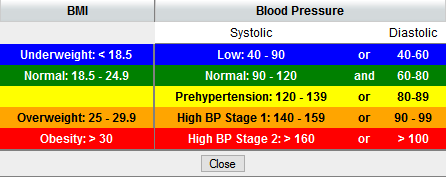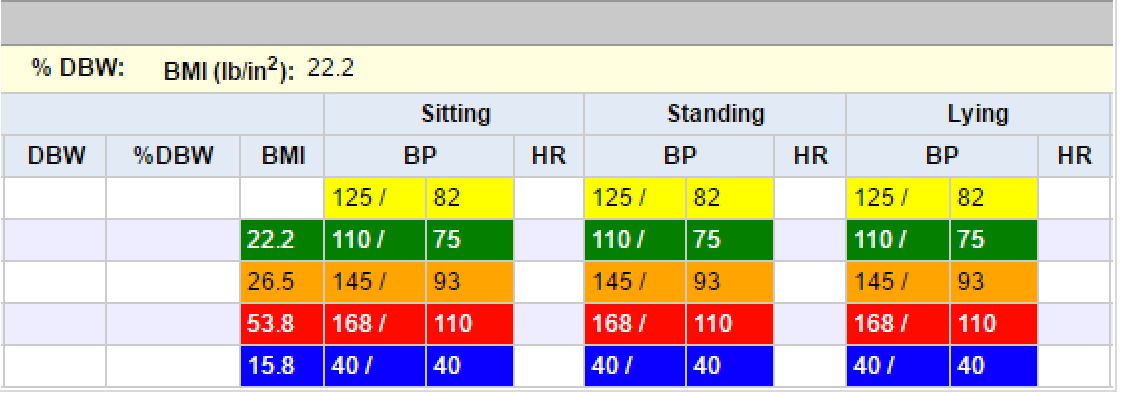Click Play button

BestNotes BMI Conversion formula:

• Calculate BMI by dividing weight in pounds (lbs) by height in inches (in) squared, and multiplying by a conversion factor of 703.
• Example: Weight = 150 lbs, Height = 5'5" (65")
• Calculation: [150 ÷ (65)2] x 703 = 24.96'

NOTE: BestNotes currently does NOT allow Vital entries larger than:

• Height: 8 feet+
• Weight (lbs): 1001+
• Temperature: 111+
• Oxygen (O2): 101+
• Respiration: 81+
• Blood Pressure (BP):
• Systolic: 201+
• Diastolic: 121+
• Heart Rate: 241+